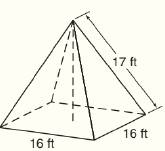Chapter 9.CT, Problem 5CTElementary Geometry For College St...

7th Edition
Alexander + 2 others
ISBN: 9781337614085

Solutions

Chapter
SectionElementary Geometry For College St...

7th Edition
Alexander + 2 others
ISBN: 9781337614085
Textbook Problem

For the regular square pyramid shown, find the length of the slant height.To determine

To find:

The slant height of the regular square pyramid.

Explanation

Given:

The regular square pyramid is shown below

Calculation:

The triangular lateral face is an isosceles triangle as shown below

Here AD is the slant height l.

By Pythagoras theorem,

Still sussing out bartleby?

Check out a sample textbook solution.

See a sample solution

The Solution to Your Study Problems

Bartleby provides explanations to thousands of textbook problems written by our experts, many with advanced degrees!

Get Started